# -The fizz produced when an alka seltzer tablet is dissolved in water is due to the...

-The fizz produced when an alka seltzer tablet is dissolved in water is due to the reaction between sodium bicarbonate NaHCo3 and Citric acid C6H8O7. What volume, in Liters, of a 2.50-M NaHCO3 solution is needed to react completely with 0.025L of a 3.00-M C6H8O7 solution? The balanced eq is 3NaHCO3+C6H8O7=3CO2+3H2O+Na3C6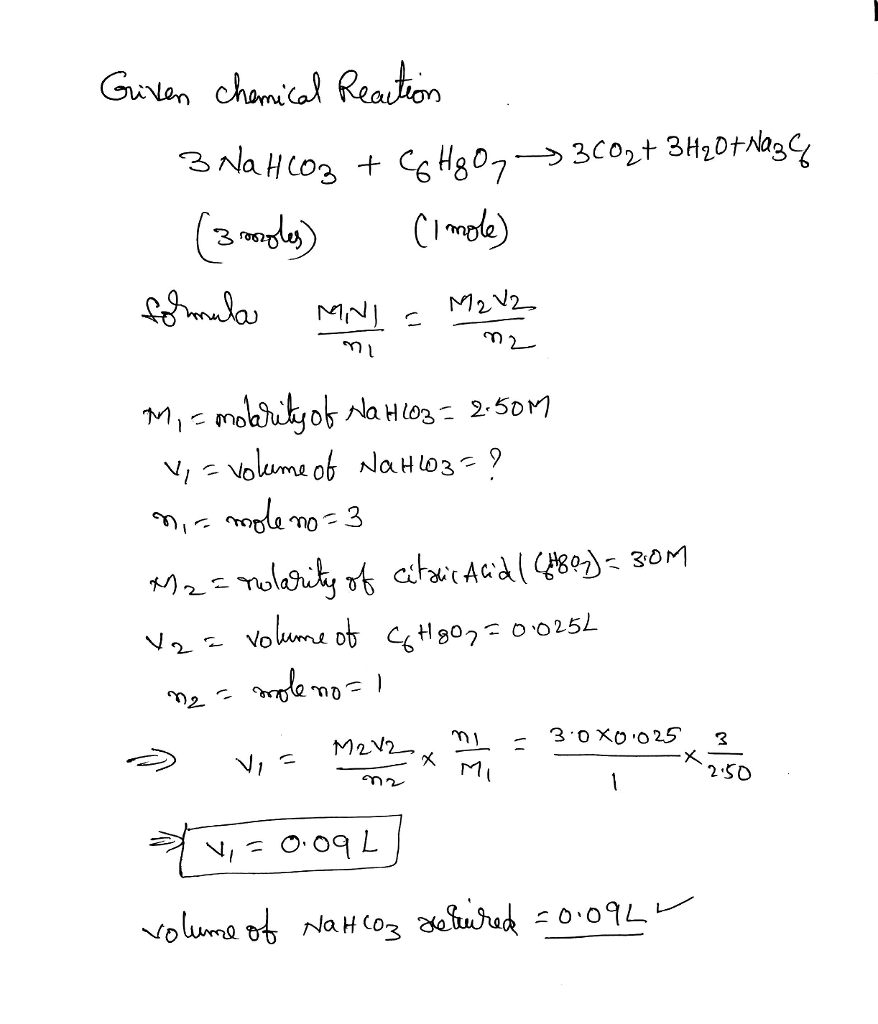##### Add Answer of: -The fizz produced when an alka seltzer tablet is dissolved in water is due to the...
Similar Homework Help Questions
• ### alka seltzer

The reaction between sodium bicarbonate (NaHCO3) and citric acid (H3C6H5O7) is as follows:3NaHCO3 (aq) + H3C6H5O7 (aq) ? 3CO2 (g) + 3H2O (l) + Na3C6H5O7 (aq)If 1.00 g sodium bicarbonate and 1.00 g citric acid are allowed to react, how many grams of carbon dioxide will form?A) 0.45B) 0.52C) 0.23D) 0.64E) 0.33

• ### limiting reageants

The fizz produced when an Alka-Seltzer® tablet is dissolved in water is due to the reaction between sodium bicarbonate (NaHCO3) and citric acid (H3C6H5O5): In acertain experiment 1.50 of sodium bicarbonate and 1.50 of citric acid are allowed to react.How many grams of carbon dioxide form?How many grams of the excess reactant remain after the limiting reactant is completely consumed?

• ### 4. Alka-Seltzer contains sodium bicarbonate (NaHCO3) and citric acid 113 CH 07. When a tablet is...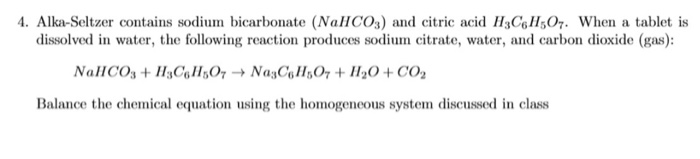4. Alka-Seltzer contains sodium bicarbonate (NaHCO3) and citric acid 113 CH 07. When a tablet is dissolved in water, the following reaction produces sodium citrate, water, and carbon dioxide (gas): NaHCO3 + H3C H50, NagC6H50- + H2O + CO2 Balance the chemical equation using the homogeneous system discussed in class

• ### An Alka-Seltzer tablet consists of NaHCOs (sodium bicarbonate), citric acid (H3C&HsO; citric acid...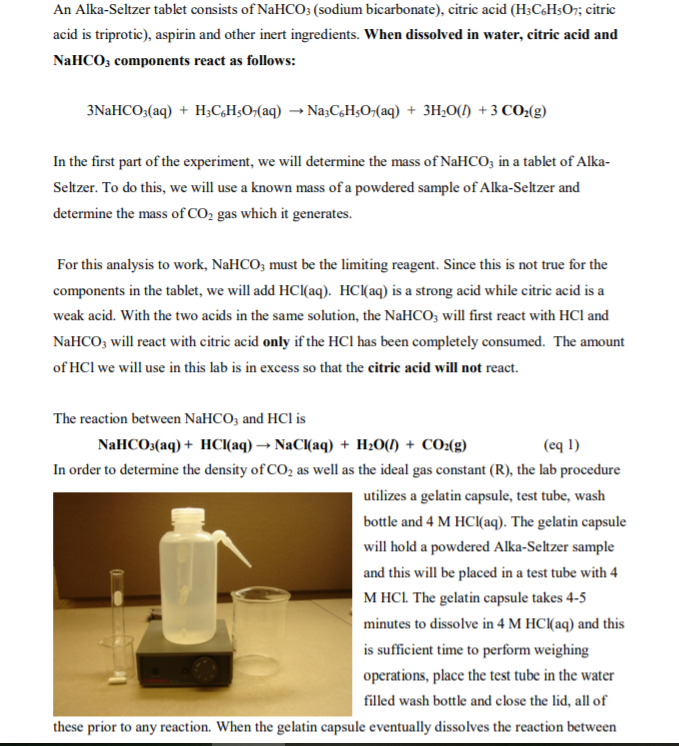I Need the pre-lab Questions, please An Alka-Seltzer tablet consists of NaHCOs (sodium bicarbonate), citric acid (H3C&HsO; citric acid is triprotic), aspirin and other inert ingredients. When dissolved in water, citric acid and NaHCO3 components react as follows: In the first part of the experiment, we will determine the mass of NaHCO in a tablet of Alka Seltzer. To do this, we will use a known mass of a powdered sample of Alka-Seltzer and determine the mass of CO2 gas...

• ### Problem 3.73

The fizz produced when an Alka-Seltzer® tablet is dissolved in water is due to the reaction between sodium bicarbonate (rm NaHCO_3) and citric acid (rmH_3C_6H_5O_7): 3{rm NaHCO_3} (aq) + {rm H_3C_6 H_5O_7} (aq) rightarrow 3{rm CO_2} (g) + 3{rm H_2} {rm O}(l) + {rm Na_3C_6H_5O_7} (aq) In a certain experiment1.15 g of sodium bicarbonate and 1.15 g of citric acid are allowed to react.Part B: How many grams of carbon dioxide form?Part C: How many grams of the excess reactant...

• ### General Chemistry Problem Need help with this example!!!

The fizz produced when an Alka-Seltzer® tabletis dissolved in water is due to the reaction between sodiumbicarbonate () and citric acid (): In a certain experiment 1.30 of sodium bicarbonate and 1.30 of citric acid are allowed to react.a.) Which is the limiting reactant? Sodium bicarbonate orcitric acid?b.) How many grams of carbon dioxide form?c.) How many grams of the excess reactant remain after thelimiting reactant is completely consumed?

• ### Help me verify the solution to question #3 which includes a, b, and c. abery angery...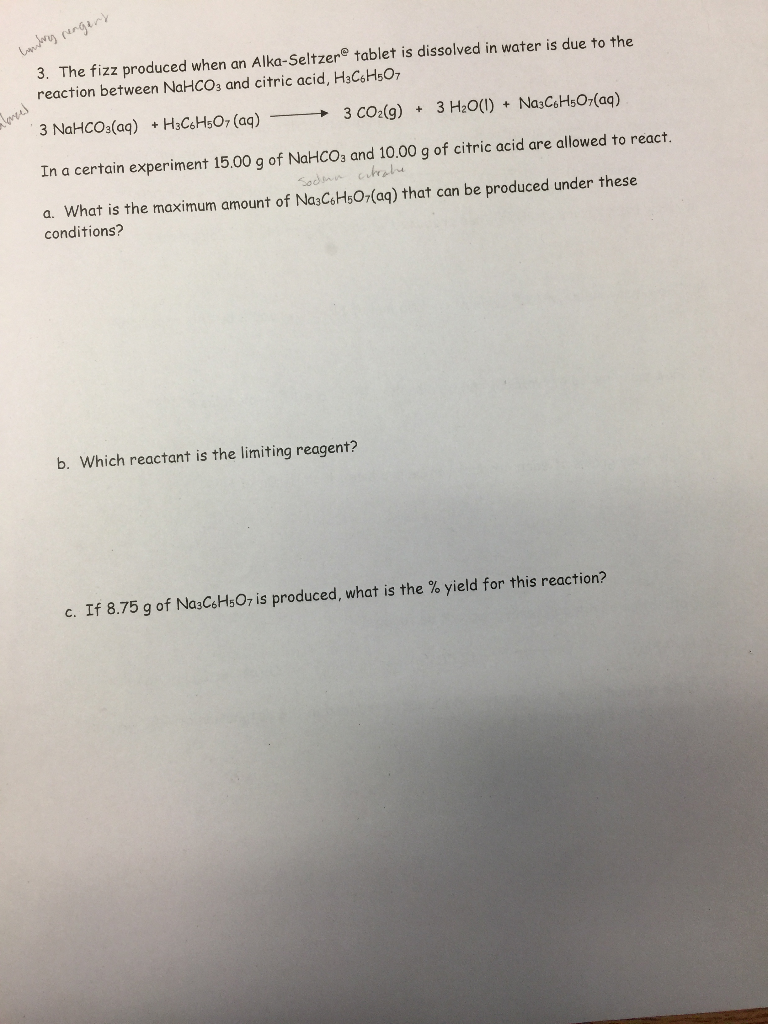Help me verify the solution to question #3 which includes a, b, and c. abery angery 3. The fizz produced when an Alka-Seltzere tablet is dissolved in water is due to the reaction between NaHCO3 and citric acid, H3C6H507 3 NaHCO3(aq) +H3CeHsO7 (aq) 3 CO2(g) 3 H20(I) Na3CeHs O7(aq) In a certain experiment 15.00 g of NaHCO3 and 10.00 g of citric acid are allowed to react. Sodmn cra a. What is the maximum amount of Na3C6H5O7(aq) that can be...

• ### 124 2. Write the balan 2. Write the balanced total reaction that would occur w neutralizes...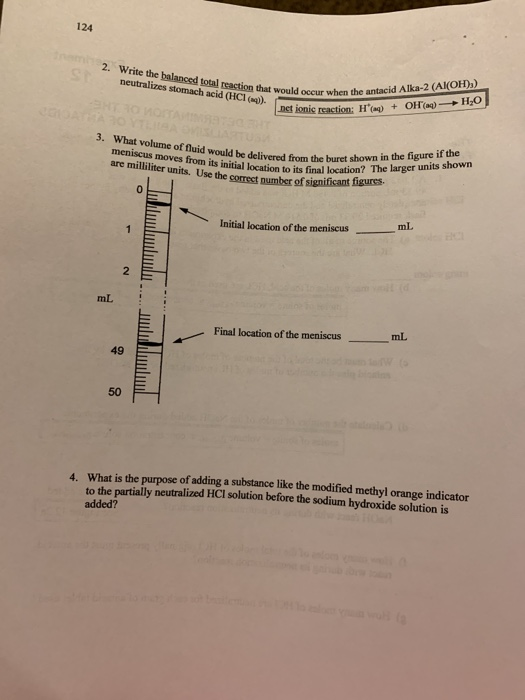124 2. Write the balan 2. Write the balanced total reaction that would occur w neutralizes stomach acid (HCl(). occur when the antacid Alka-2 (Al(OH):) bet ionic reaction: H ) + OH(aq) - H30 3. What volume of fluid would be delivered from the bure meniscus moves from its initial location to its final location are milliliter units. Use the correct number of significant 118 ered from the buret shown in the figure if the on to its final location?...

• ### Help with any of these:    21

Help with any of these:    21. Aluminium sulphate is often used by gardeners to acidify soil and can be produced by the reaction 2Al(OH)3(aq) + 3 H2SO4(aq) à Al2(SO4)3(aq)  + 6 H2O(l) The volume of 0.275 mol/L H2SO4(aq) needed to react completely with 112 mL of 0.355 mol/l Al(OH)3(aq) is A. 96.4 mL B. 145 mL C. 168 mL D. 217 mL   22. To produce the anti cancer drug cisplatin (Pt (NH3)2Cl2(s)), a technician reacted NH3(aq) with excess K2PtCl4(aq). The...

• ### [1pt] The barometric pressure measured outside an airplaneat 8 km (26248 ft) was 348 mmHg

1. [1pt] The barometric pressure measured outside an airplaneat 8 km (26248 ft) was 348 mmHg. Calculate the pressurein kPa. Do not enter unit. 2. [1pt] If 637 dm3 of krypton at 218 kPa and 18C is compressedinto a 28.0-dm3 tank at the same temperature, what isthe pressure (in kPa) of krypton in the tank? Enter unit. 3. [1pt] A sample of 70.0 cm3 of argon gas at 19C wascontained at a pressure of 198 kPa in a J-shaped tube withmercury. Later...

Need Online Homework Help?